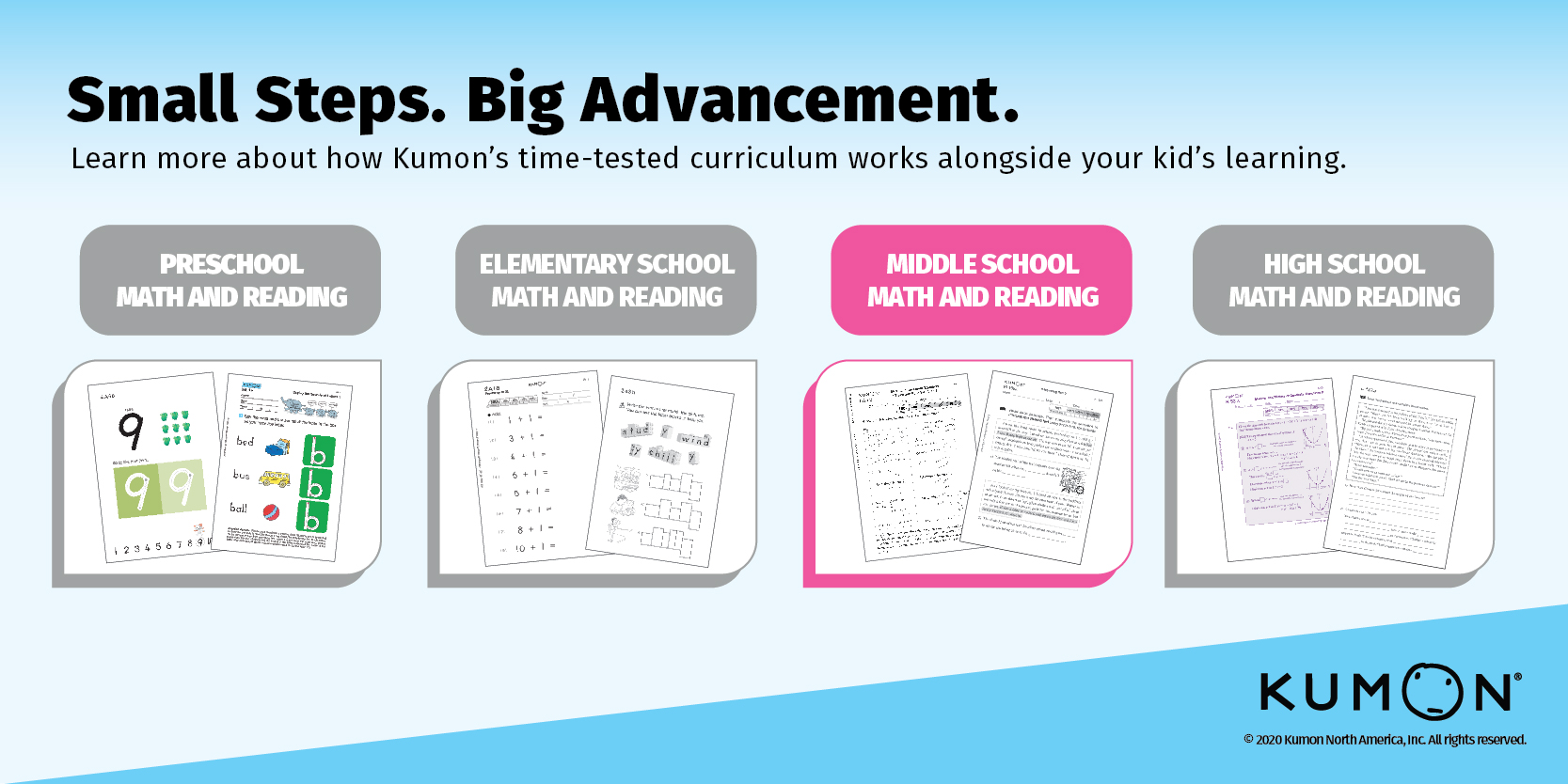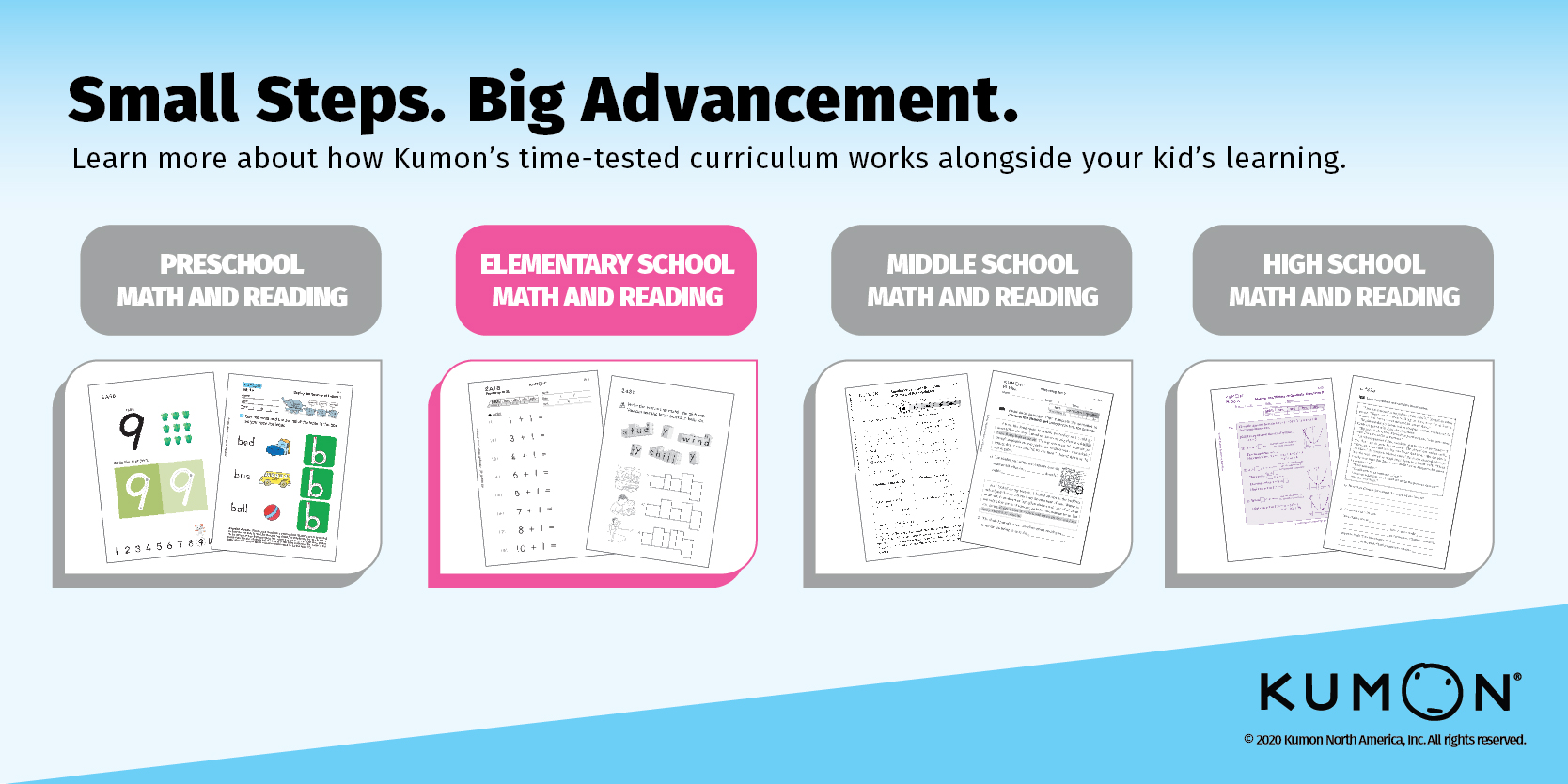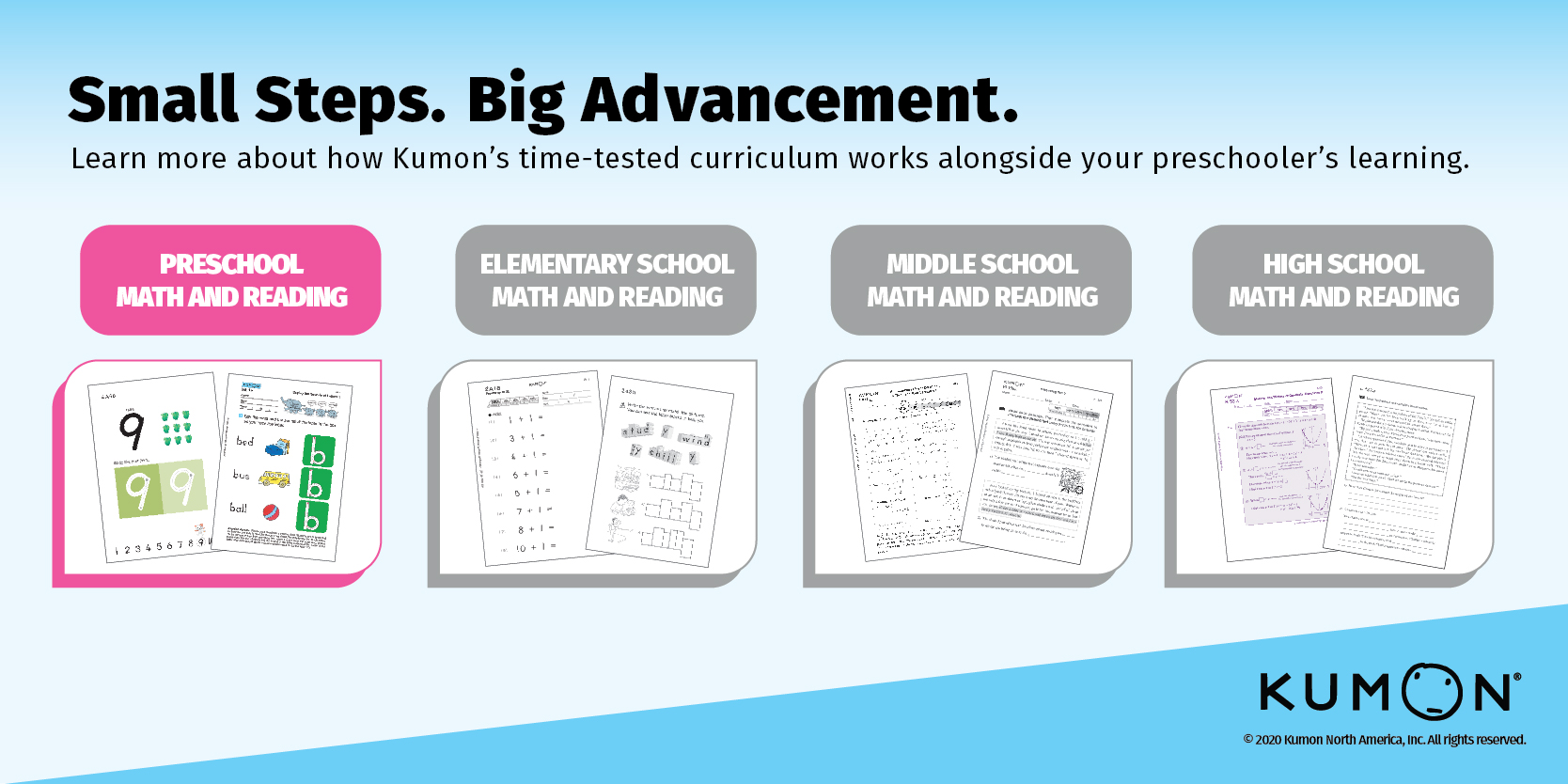Kumon's Curriculum Series Archives - Kumon

# Kumon’s Curriculum Series Tips### How Kumon’s Curriculum Supports High School Learning

High School Math

In the levels of the Kumon Math Program that correspond with high school learning, students fully develop their algebraic skills and progress to precalculus, trigonometry, and ultimately calculus. They will study concepts like functions, graphs, logarithms, sequences, and series. When students reach the final level of### How Kumon’s Curriculum Supports Middle School Learning

Middle School Math

In the levels of the Kumon Math Program that correspond with middle school, students become more familiar with fractions and decimals by using them in word problems and calculating positive and negative numbers. They are introduced to algebraic expressions before### How Kumon’s Curriculum Supports Elementary School Learning

Elementary School Math

In the levels of the Kumon Math Program that correspond with elementary school, students deepen their understanding of the four basic operations: addition, subtraction, multiplication and division. Students get started with single digits and progress to challenging problems as they### How Kumon’s Curriculum Supports Your Preschoolers’ Learning

Preschool Math

In the levels of the Kumon Math Program that correspond with
preschool learning, students deepen their understanding of the number sequence,
helping them develop the skills they’ll need when they learn addition and
subtraction. Students first learn to recite and write